# Punjab Board (PSEB) Question Paper for Class 10th Maths 2019 In PDF

## PSEB Matriculation (10th Standard) Maths Exam Question Papers 2019 with Solutions – Free Download

PSEB (Punjab board) Class 10 Maths 2019 Question Paper with solutions are available at BYJU’S in a pdf format that can be downloaded at any time for free. Mathematics is a scoring subject for the students among all the subjects of Class 10. Students can score full marks in PSEB Class 10 Maths exam if they are thorough with all the concepts of Class 10 Maths. Practice of all the previous papers of PSEB Class 10 Maths as it help in understanding the exam pattern. This in turn helps in solving various types of questions that occur in the annual exam of Punjab board. Students can solve Punjab Board (PSEB) Question Paper for Class 10th Maths 2019 In PDF with Solutions to get practice and ace the exams.

### PSEB Class 10 Mathematics 2019 Question Paper with Solutions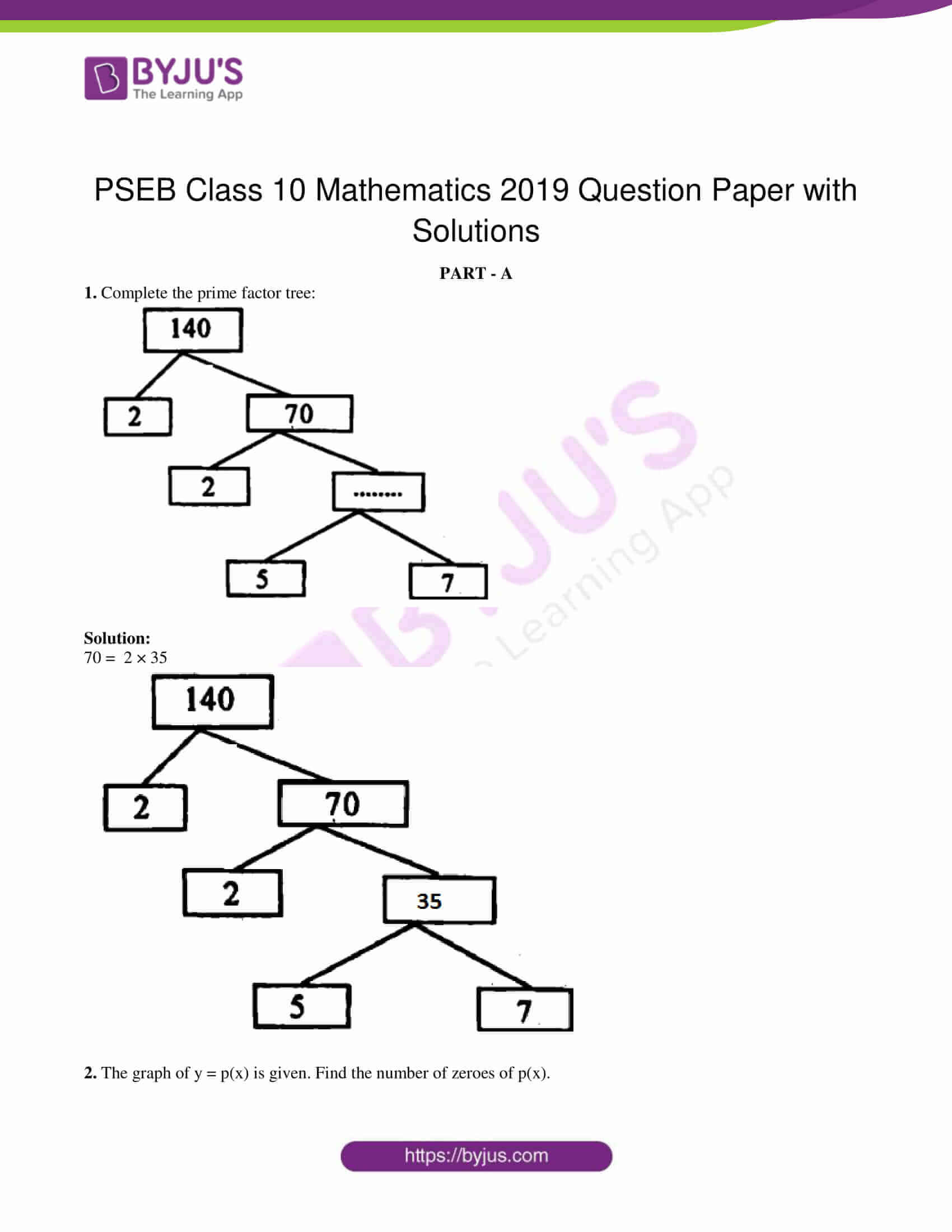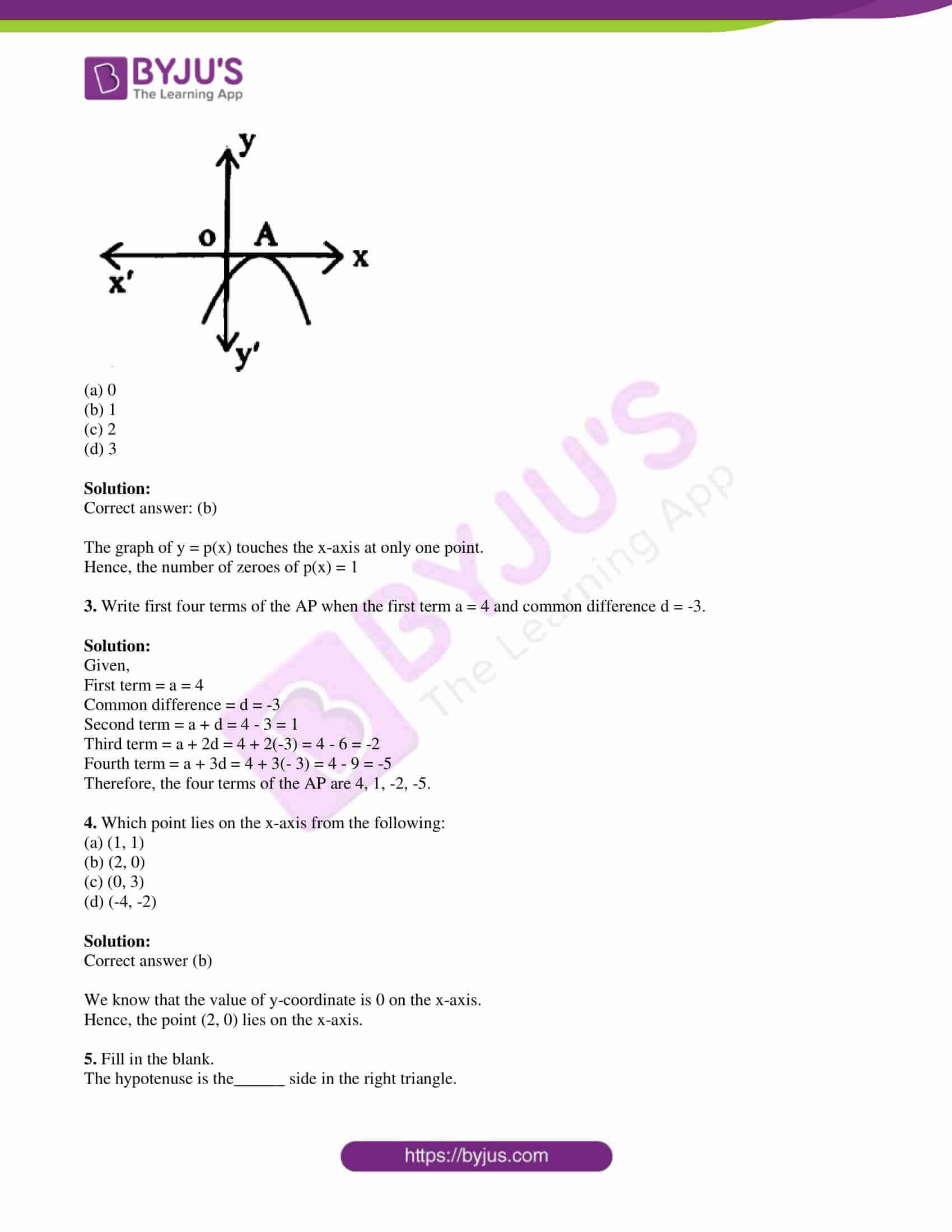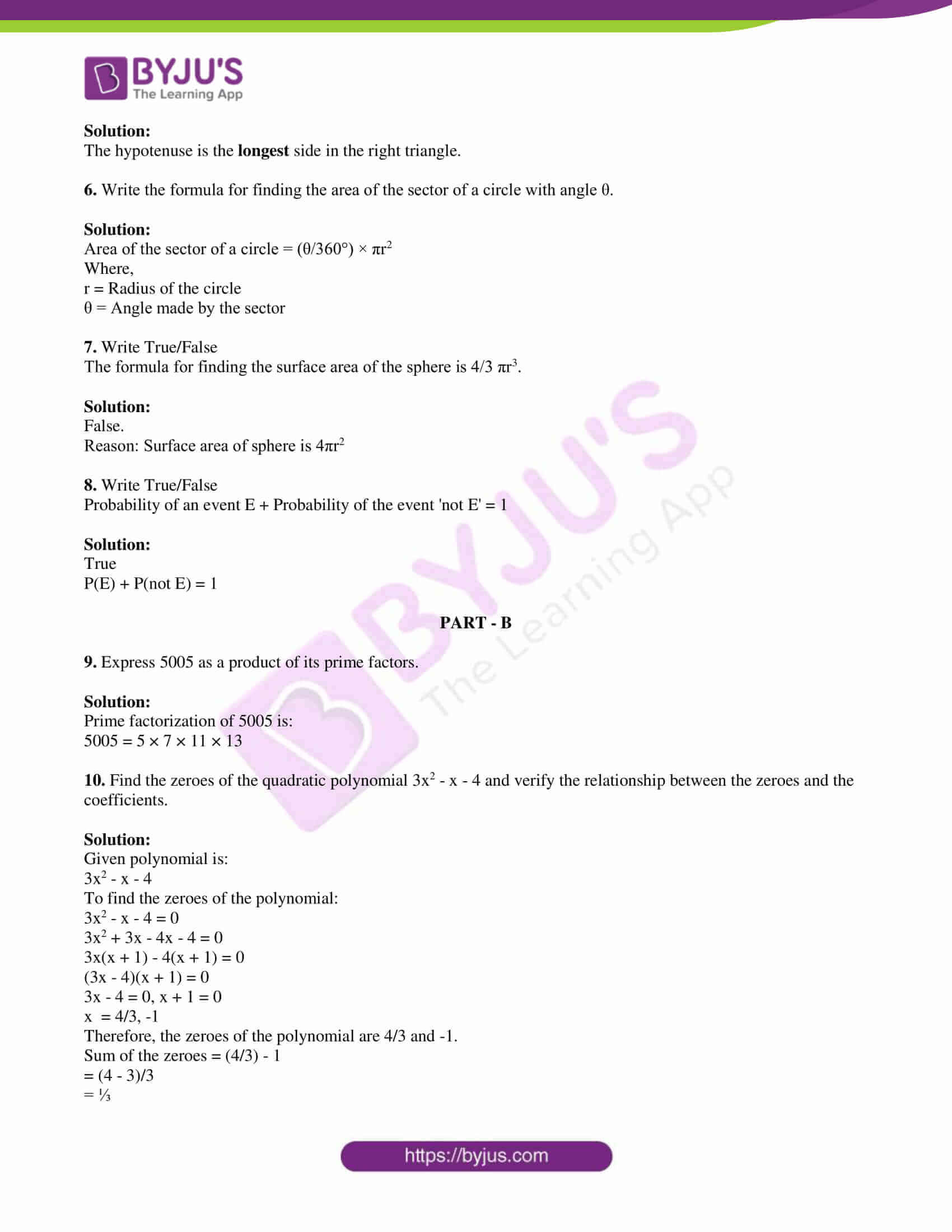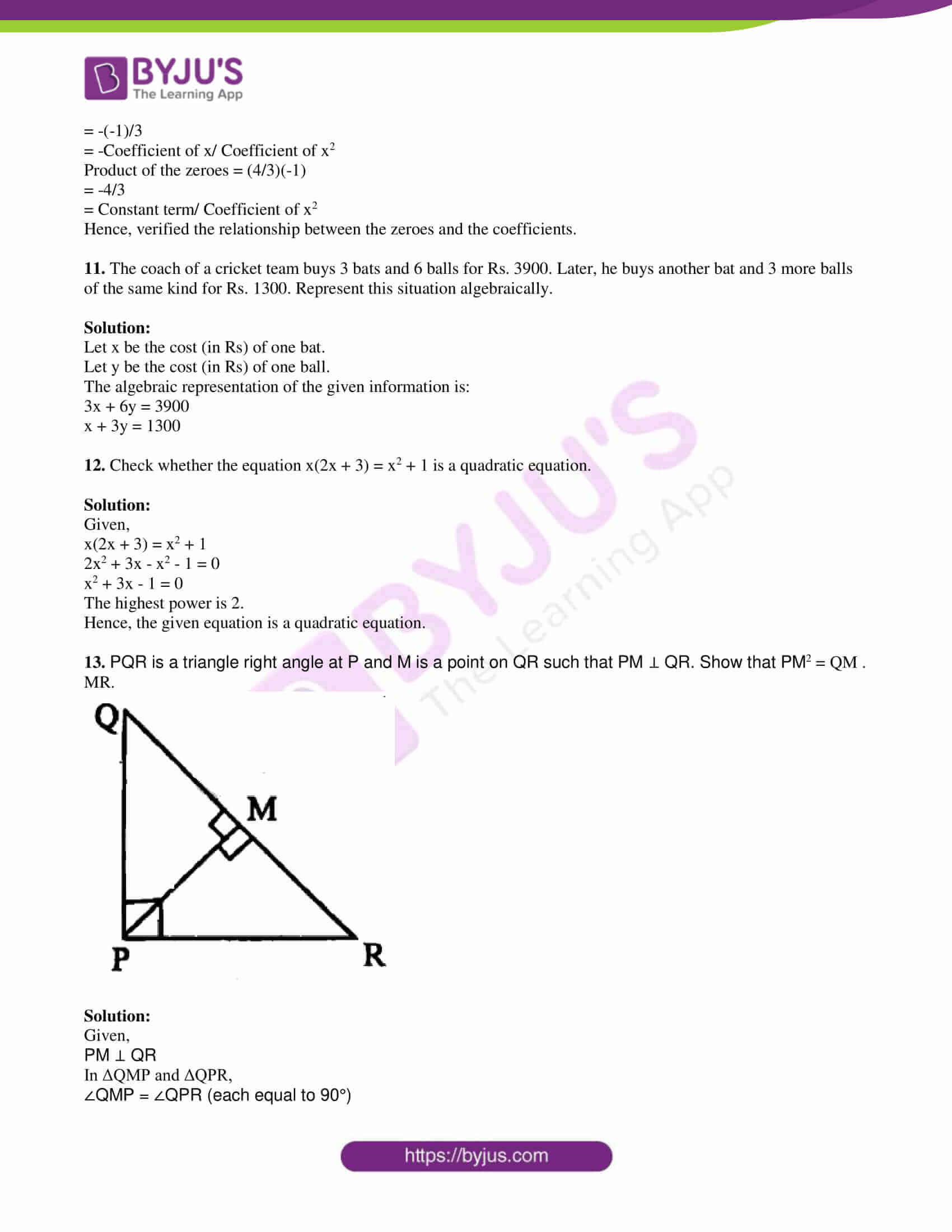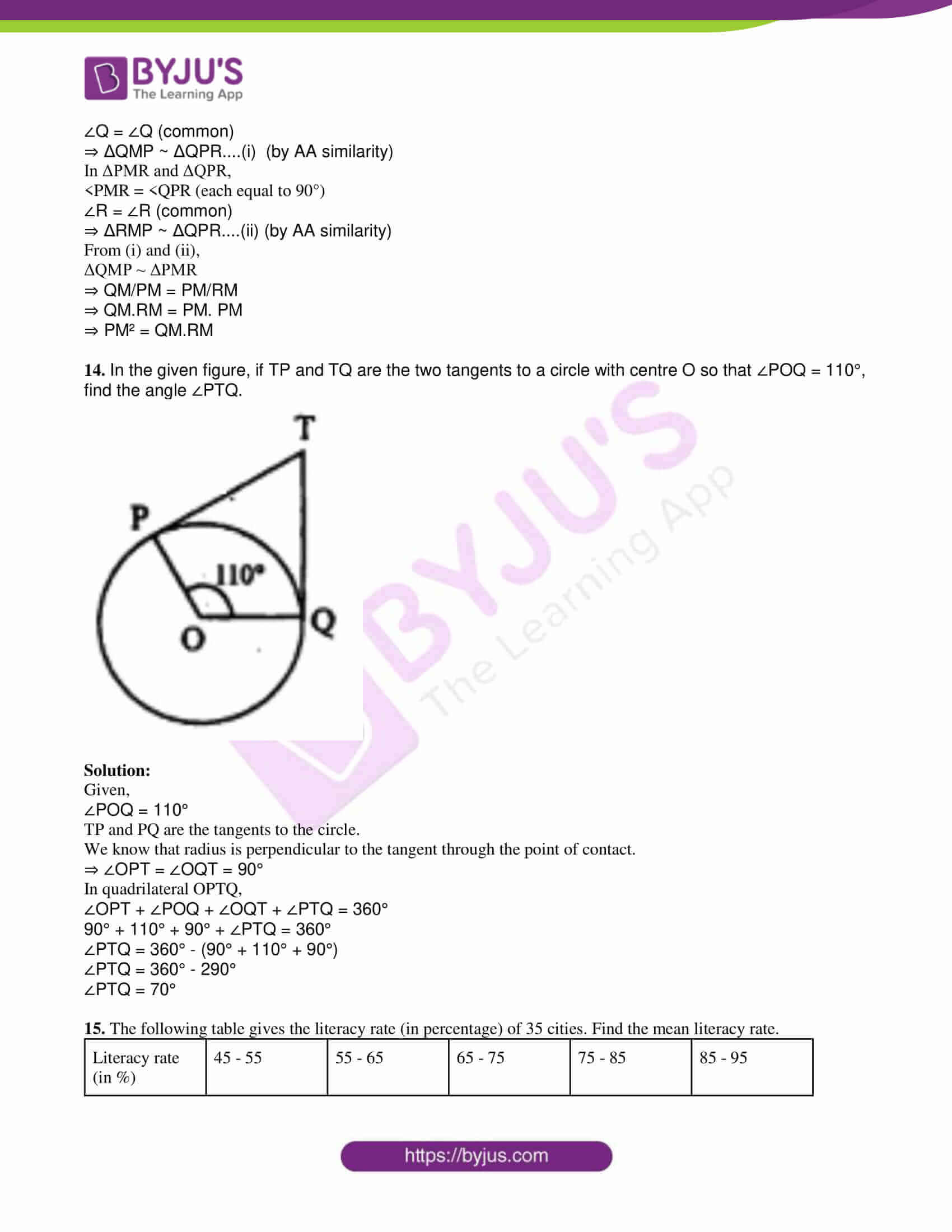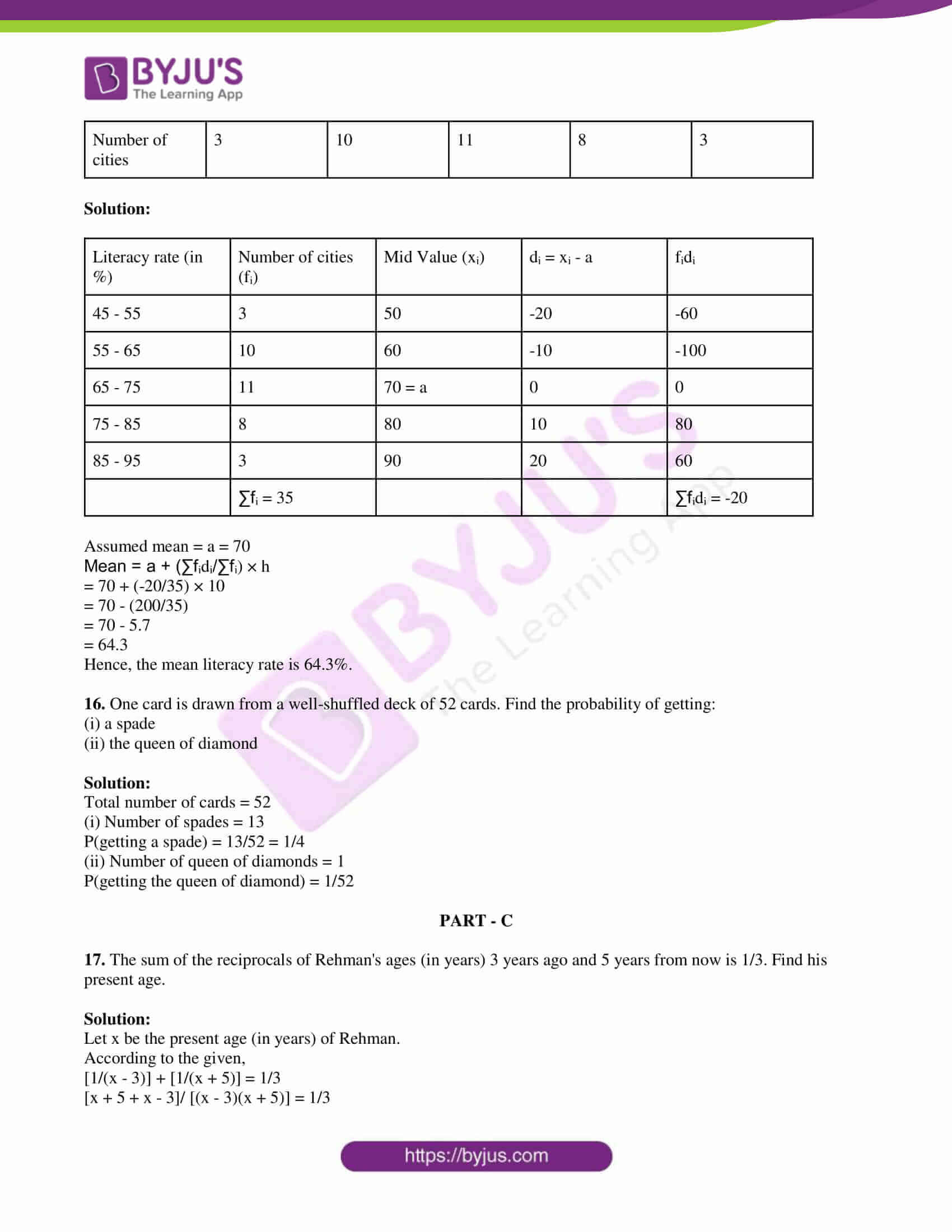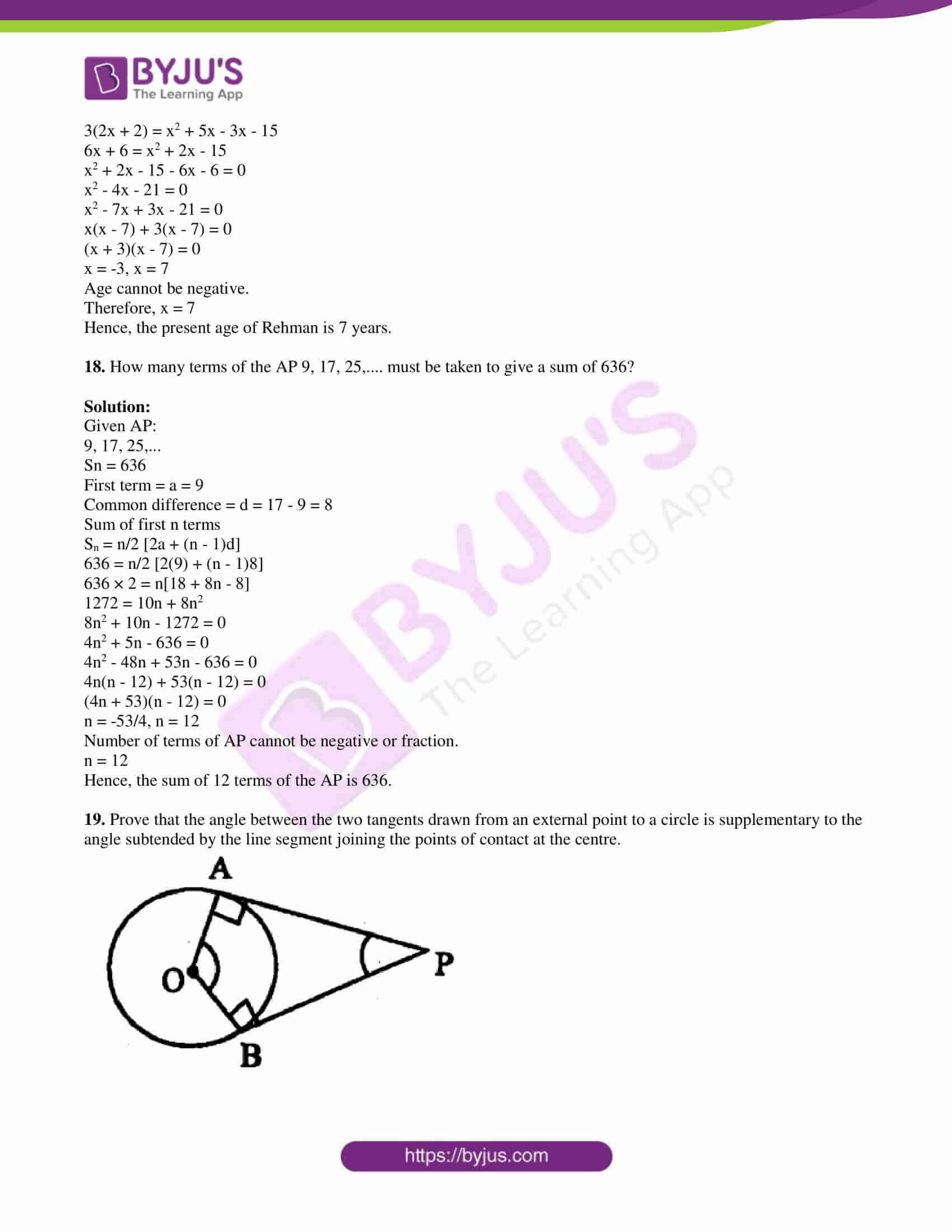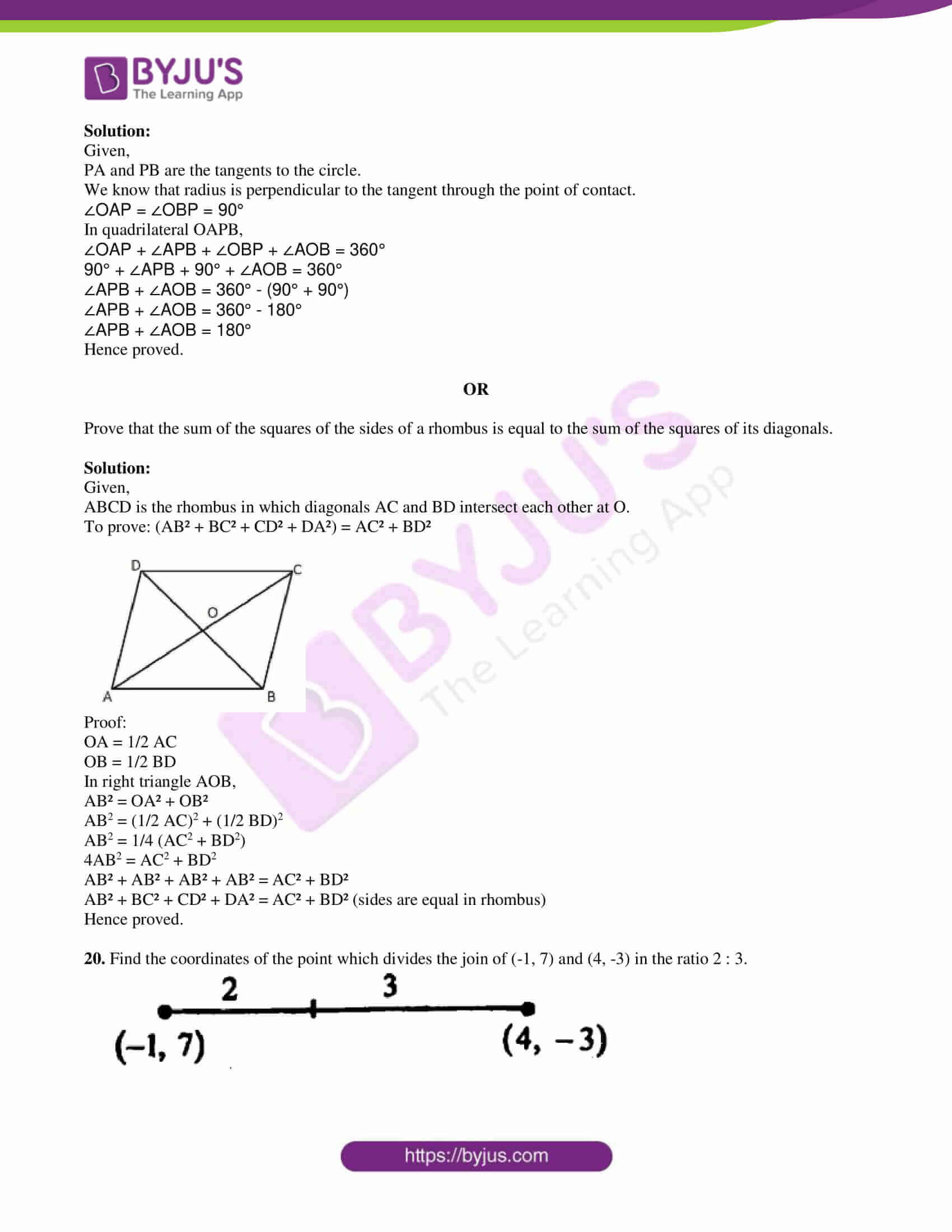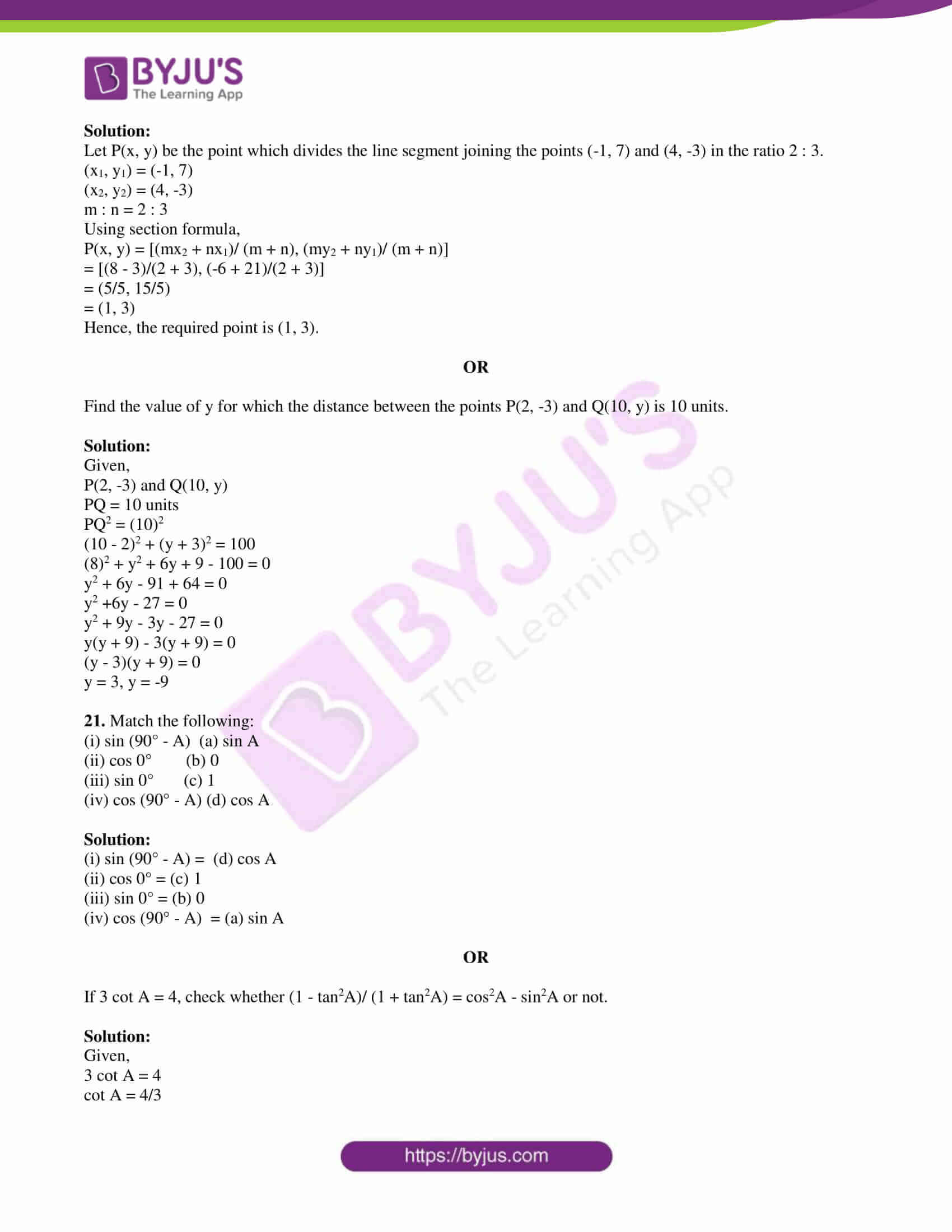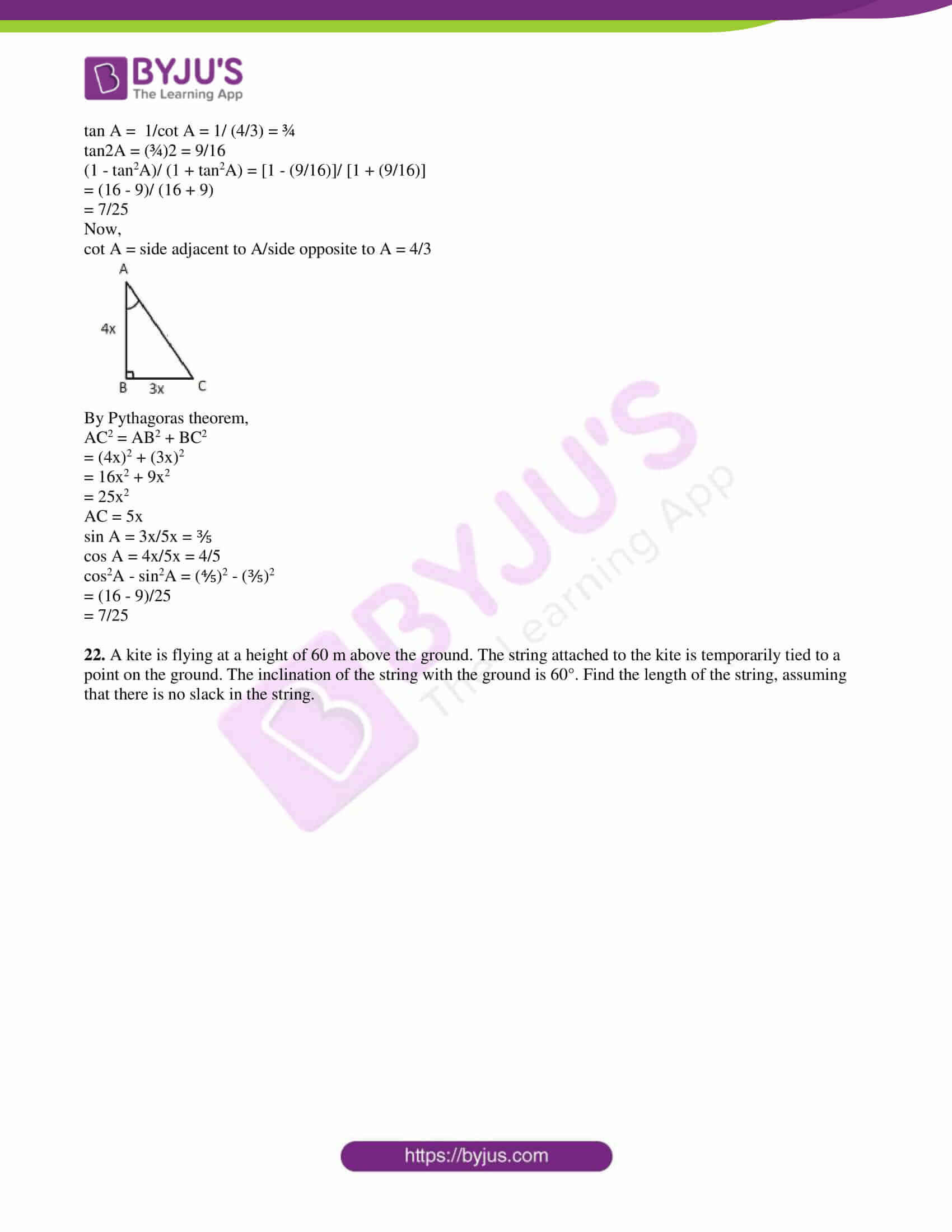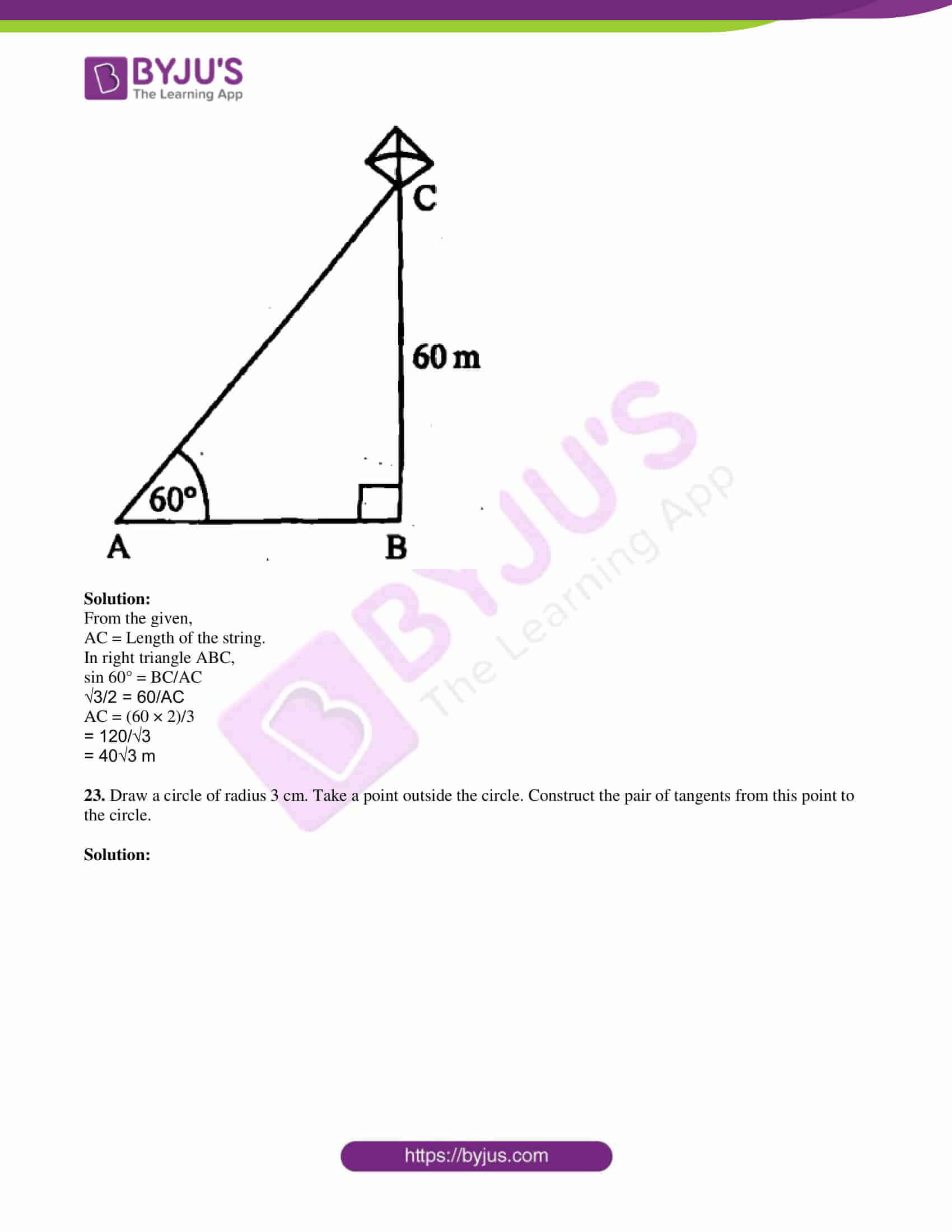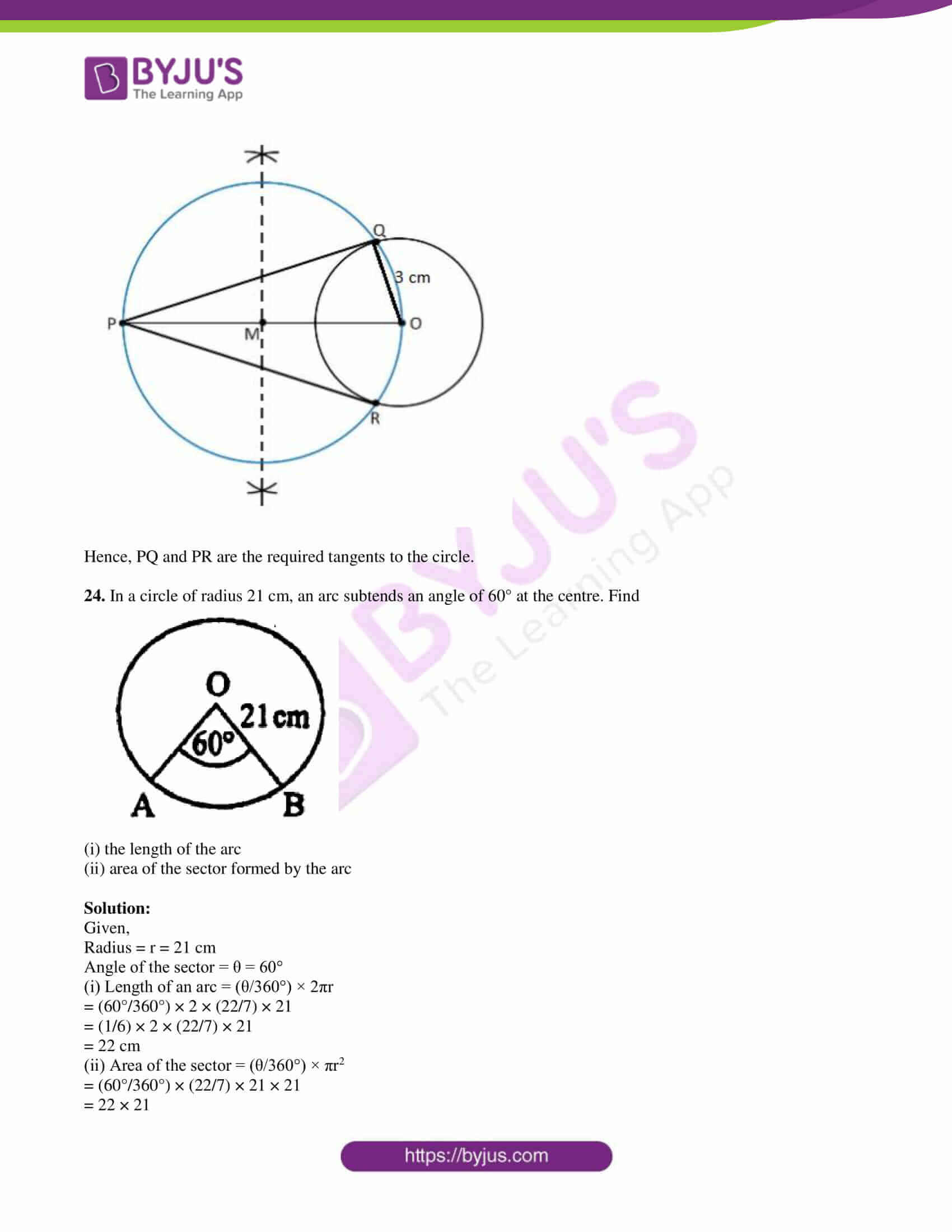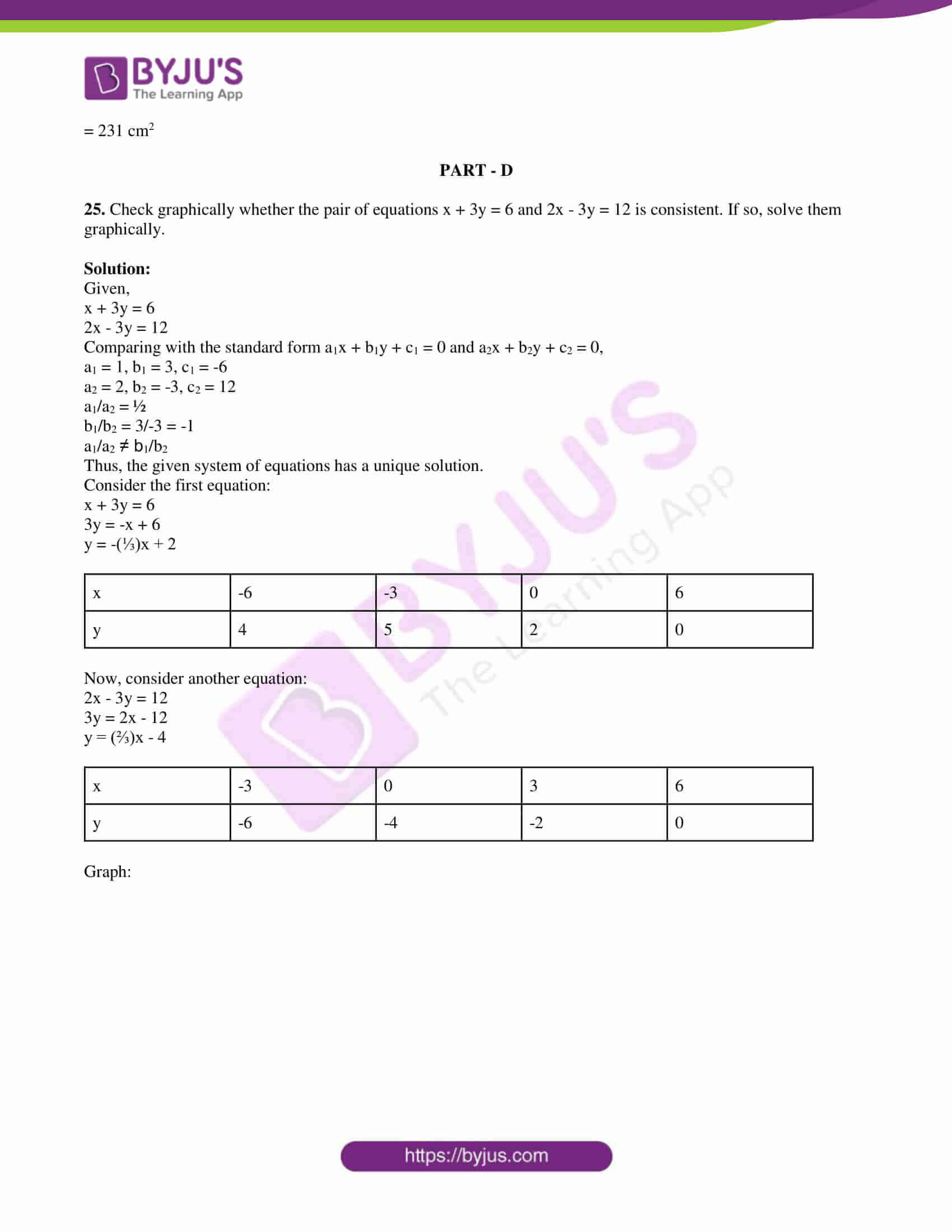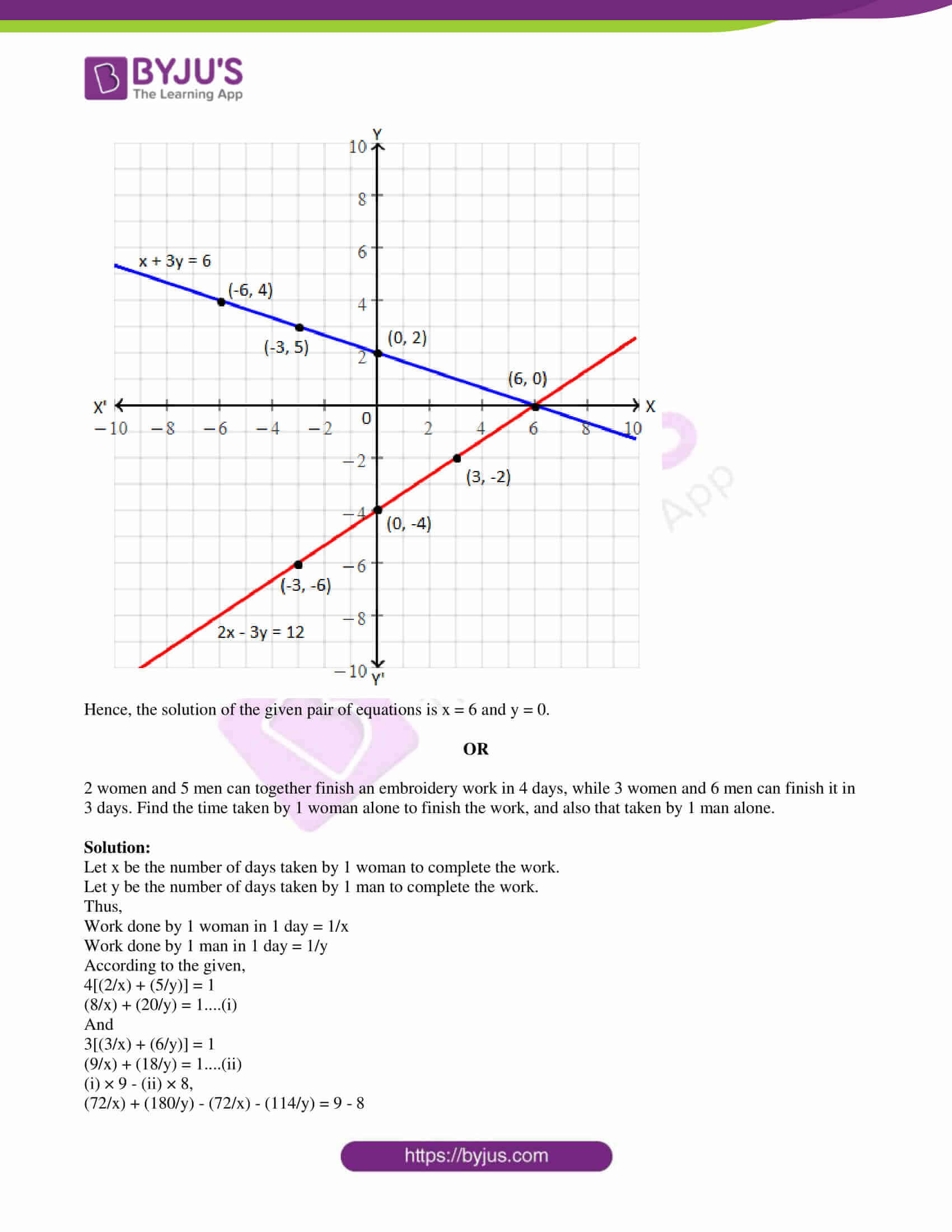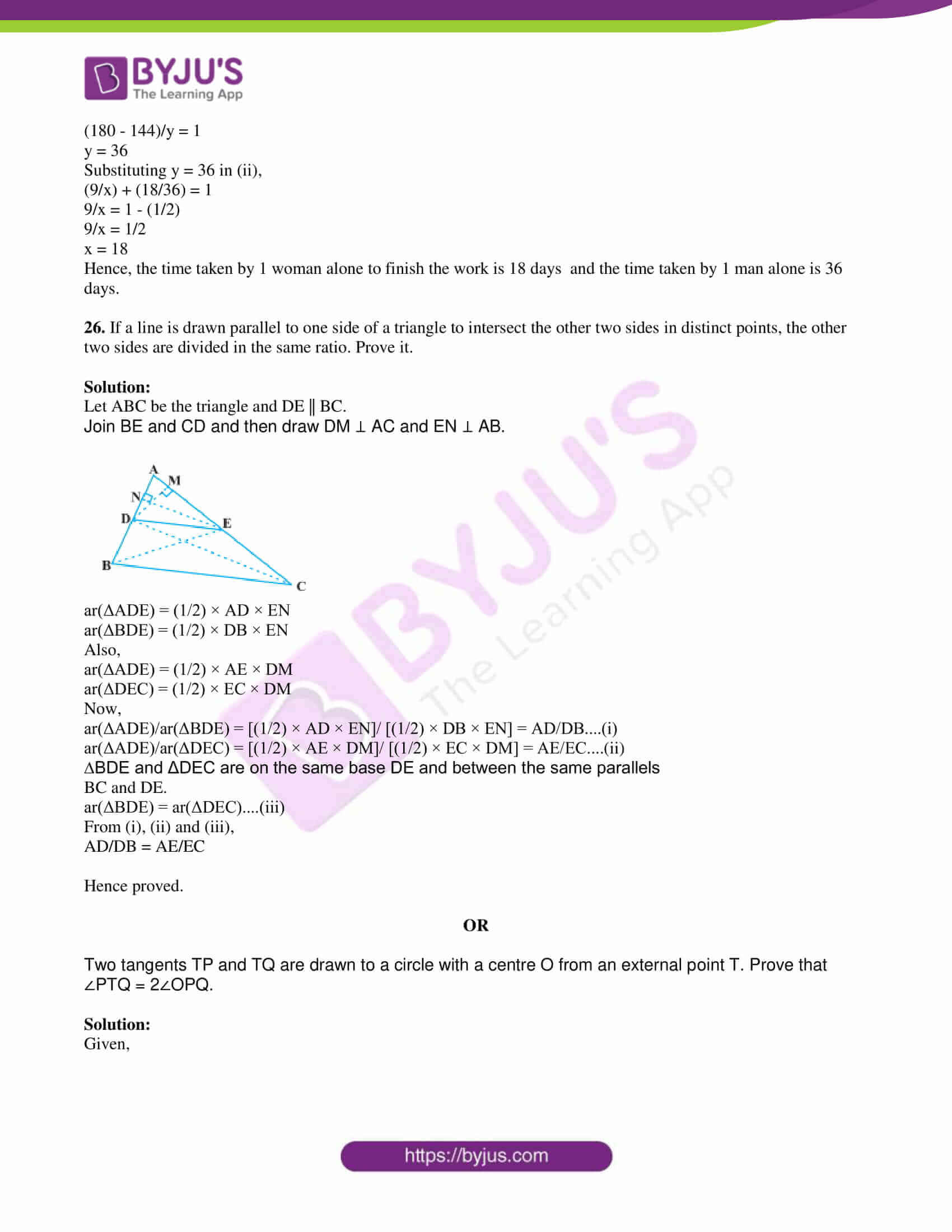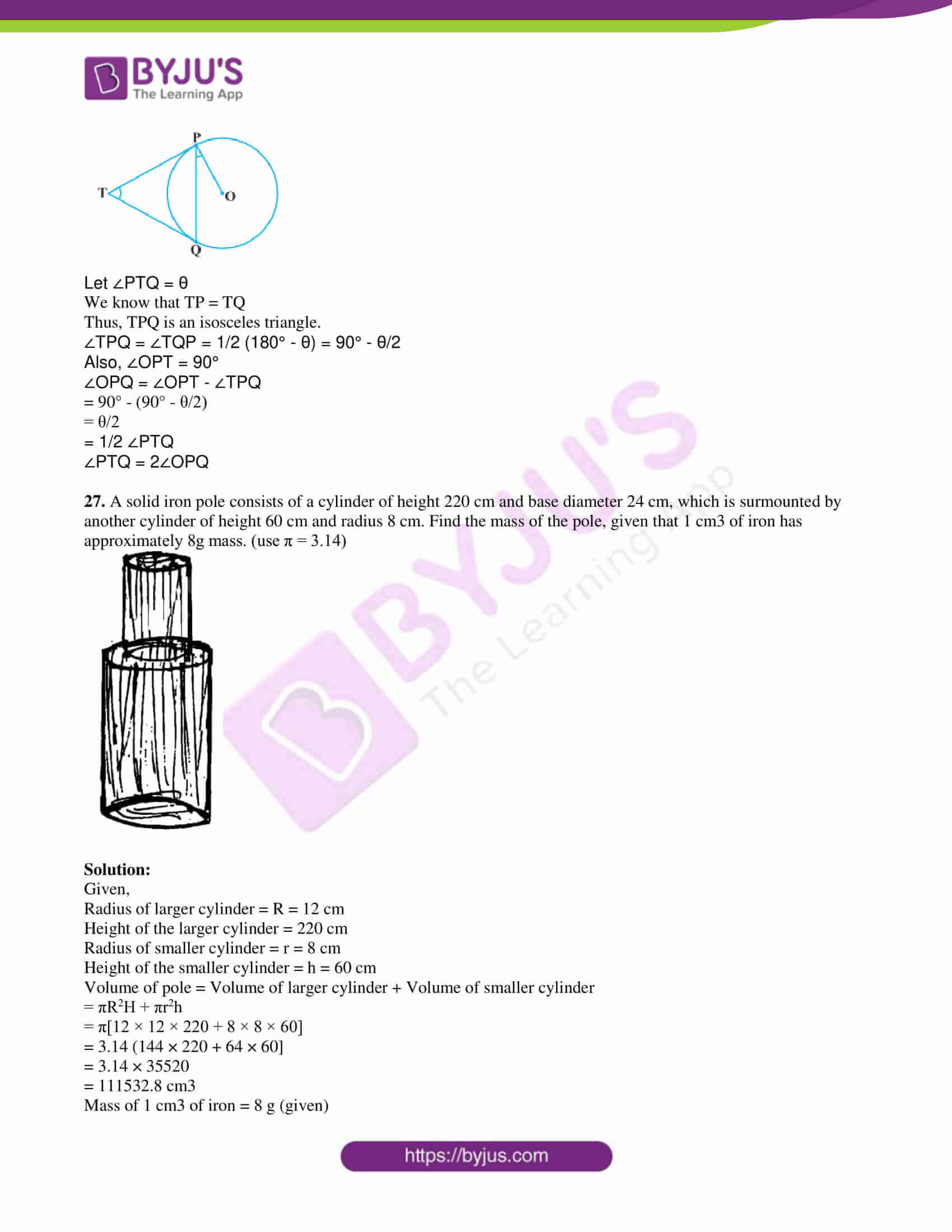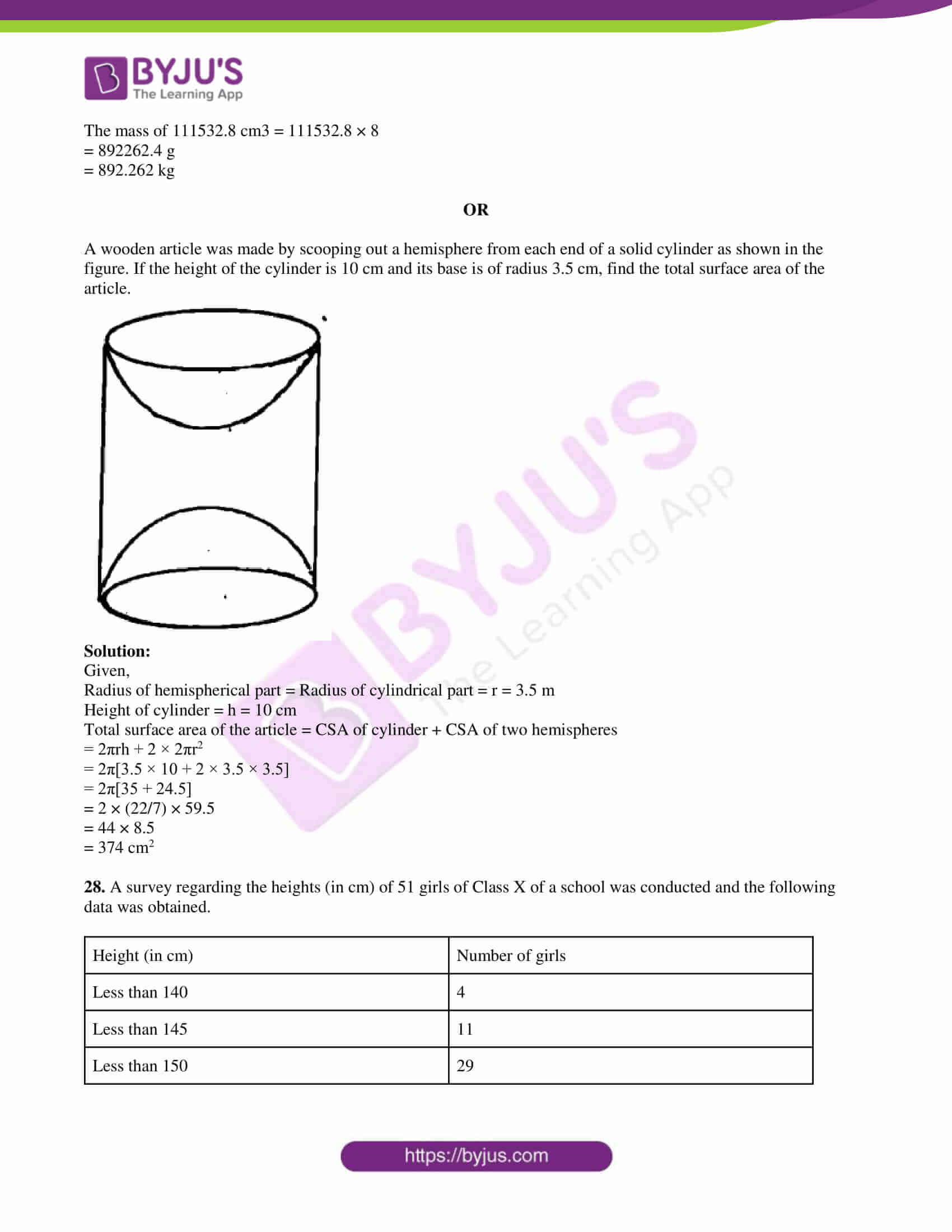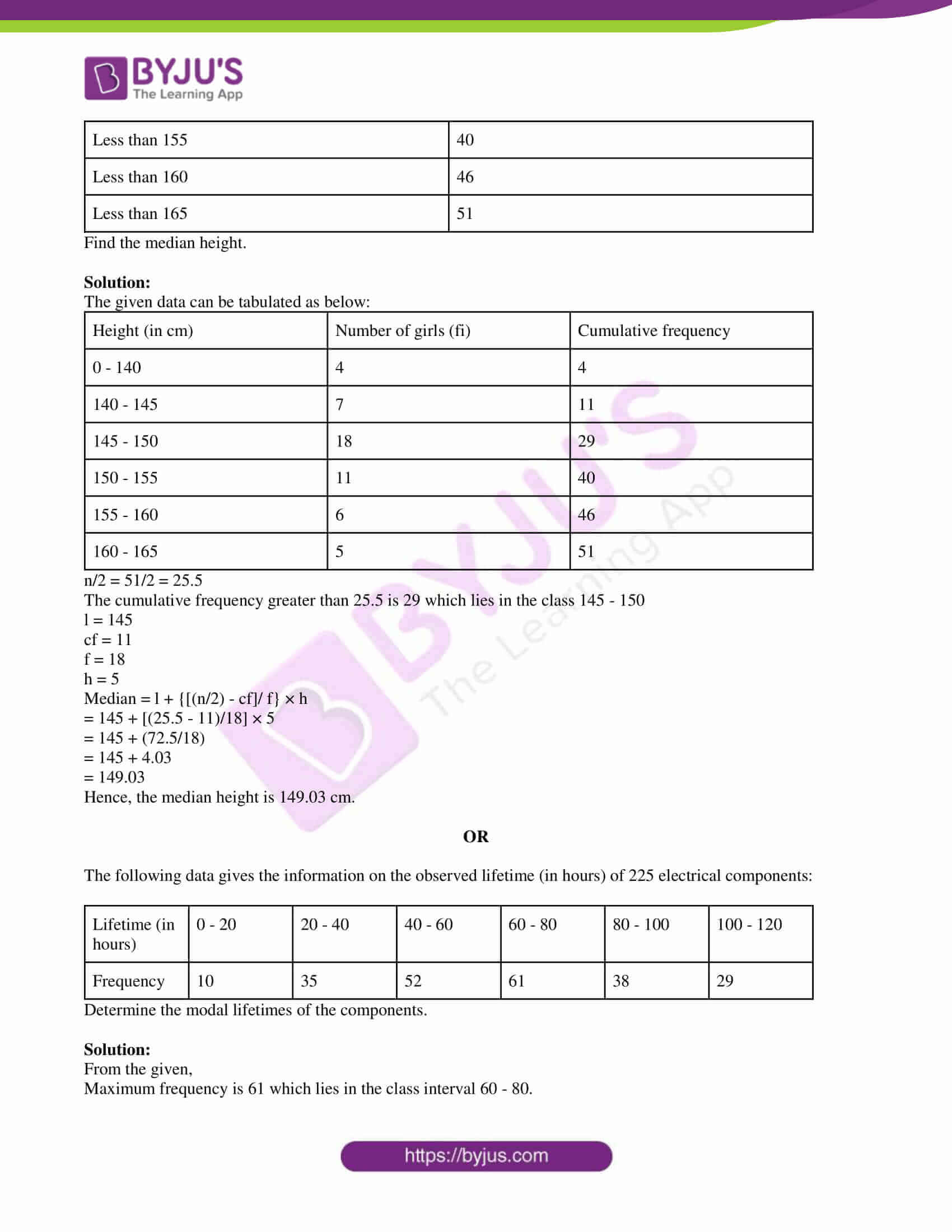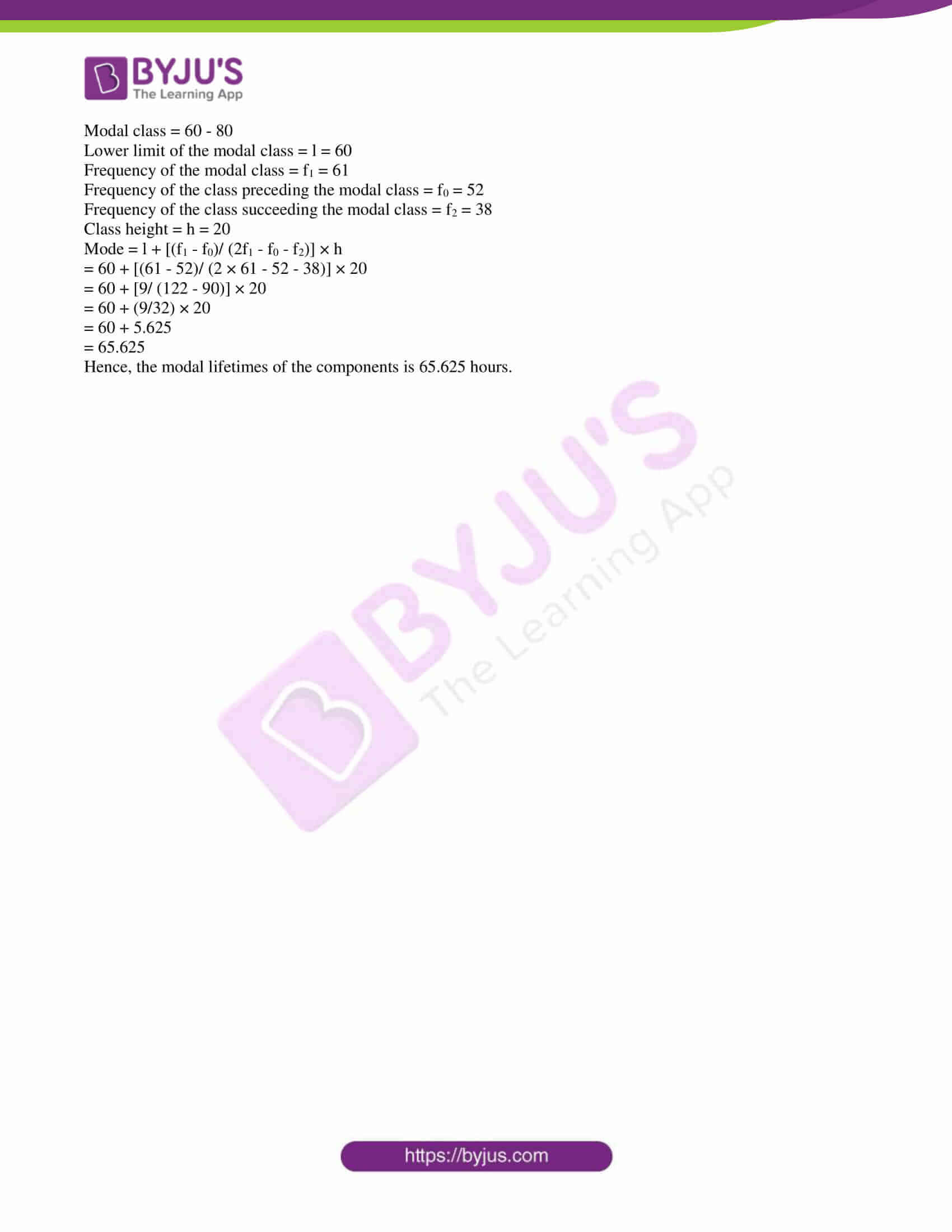PART – A

1. Complete the prime factor tree: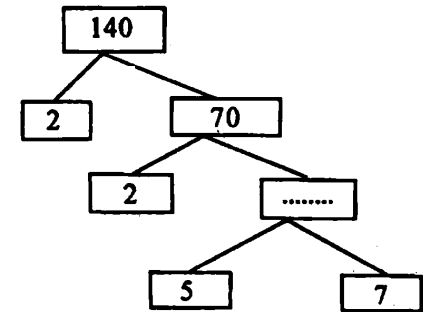Solution:

70 = 2 × 35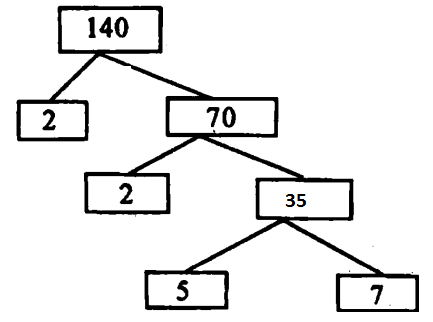2. The graph of y = p(x) is given. Find the number of zeroes of p(x).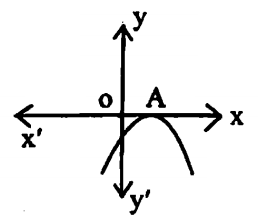(a) 0

(b) 1

(c) 2

(d) 3

Solution:

The graph of y = p(x) touches the x-axis at only one point.

Hence, the number of zeroes of p(x) = 1

3. Write first four terms of the AP when the first term a = 4 and common difference d = -3.

Solution:

Given,

First term = a = 4

Common difference = d = -3

Second term = a + d = 4 – 3 = 1

Third term = a + 2d = 4 + 2(-3) = 4 – 6 = -2

Fourth term = a + 3d = 4 + 3(- 3) = 4 – 9 = -5

Therefore, the four terms of the AP are 4, 1, -2, -5.

4. Which point lies on the x-axis from the following:

(a) (1, 1)

(b) (2, 0)

(c) (0, 3)

(d) (-4, -2)

Solution:

We know that the value of y-coordinate is 0 on the x-axis.

Hence, the point (2, 0) lies on the x-axis.

5. Fill in the blank.

The hypotenuse is the______ side in the right triangle.

Solution:

The hypotenuse is the longest side in the right triangle.

6. Write the formula for finding the area of the sector of a circle with angle θ.

Solution:

Area of the sector of a circle = (θ/360°) × πr2

Where,

r = Radius of the circle

θ = Angle made by the sector

7. Write True/False

The formula for finding the surface area of the sphere is 4/3 πr3.

Solution:

False.

Reason: Surface area of sphere is 4πr2

8. Write True/False

Probability of an event E + Probability of the event ‘not E’ = 1

Solution:

True

P(E) + P(not E) = 1

PART – B

9. Express 5005 as a product of its prime factors.

Solution:

Prime factorization of 5005 is:

5005 = 5 × 7 × 11 × 13

10. Find the zeroes of the quadratic polynomial 3x2 – x – 4 and verify the relationship between the zeroes and the coefficients.

Solution:

Given polynomial is:

3x2 – x – 4

To find the zeroes of the polynomial:

3x2 – x – 4 = 0

3x2 + 3x – 4x – 4 = 0

3x(x + 1) – 4(x + 1) = 0

(3x – 4)(x + 1) = 0

3x – 4 = 0, x + 1 = 0

x = 4/3, -1

Therefore, the zeroes of the polynomial are 4/3 and -1.

Sum of the zeroes = (4/3) – 1

= (4 – 3)/3

= ⅓

= -(-1)/3

= -Coefficient of x/ Coefficient of x2

Product of the zeroes = (4/3)(-1)

= -4/3

= Constant term/ Coefficient of x2

Hence, verified the relationship between the zeroes and the coefficients.

11. The coach of a cricket team buys 3 bats and 6 balls for Rs. 3900. Later, he buys another bat and 3 more balls of the same kind for Rs. 1300. Represent this situation algebraically.

Solution:

Let x be the cost (in Rs) of one bat.

Let y be the cost (in Rs) of one ball.

The algebraic representation of the given information is:

3x + 6y = 3900

x + 3y = 1300

12. Check whether the equation x(2x + 3) = x2 + 1 is a quadratic equation.

Solution:

Given,

x(2x + 3) = x2 + 1

2x2 + 3x – x2 – 1 = 0

x2 + 3x – 1 = 0

The highest power is 2.

Hence, the given equation is a quadratic equation.

13. PQR is a triangle right angle at P and M is a point on QR such that PM ⊥ QR. Show that PM2 = QM . MR.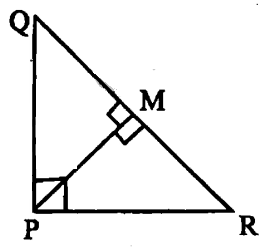Solution:

Given,

PM ⊥ QR

In ΔQMP and ΔQPR,

∠QMP = ∠QPR (each equal to 90°)

∠Q = ∠Q (common)

⇒ ΔQMP ~ ΔQPR….(i) (by AA similarity)

In ΔPMR and ΔQPR,

<PMR = <QPR (each equal to 90°)

∠R = ∠R (common)

⇒ ΔRMP ~ ΔQPR….(ii) (by AA similarity)

From (i) and (ii),

ΔQMP ~ ΔPMR

⇒ QM/PM = PM/RM

⇒ QM.RM = PM. PM

⇒ PM² = QM.RM

14. In the given figure, if TP and TQ are the two tangents to a circle with centre O so that ∠POQ = 110°, find the angle ∠PTQ.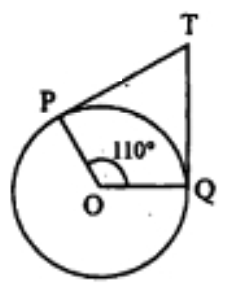Solution:

Given,

∠POQ = 110°

TP and PQ are the tangents to the circle.

We know that radius is perpendicular to the tangent through the point of contact.

⇒ ∠OPT = ∠OQT = 90°

∠OPT + ∠POQ + ∠OQT + ∠PTQ = 360°

90° + 110° + 90° + ∠PTQ = 360°

∠PTQ = 360° – (90° + 110° + 90°)

∠PTQ = 360° – 290°

∠PTQ = 70°

15. The following table gives the literacy rate (in percentage) of 35 cities. Find the mean literacy rate.

 Literacy rate (in %) 45 – 55 55 – 65 65 – 75 75 – 85 85 – 95 Number of cities 3 10 11 8 3

Solution:

 Literacy rate (in %) Number of cities (fi) Mid Value (xi) di = xi – a fidi 45 – 55 3 50 -20 -60 55 – 65 10 60 -10 -100 65 – 75 11 70 = a 0 0 75 – 85 8 80 10 80 85 – 95 3 90 20 60 ∑fi = 35 ∑fidi = -20

Assumed mean = a = 70

Mean = a + (∑fidi/∑fi) × h

= 70 + (-20/35) × 10

= 70 – (200/35)

= 70 – 5.7

= 64.3

Hence, the mean literacy rate is 64.3%.

16. One card is drawn from a well-shuffled deck of 52 cards. Find the probability of getting:

(ii) the queen of diamond

Solution:

Total number of cards = 52

(i) Number of spades = 13

P(getting a spade) = 13/52 = 1/4

(ii) Number of queen of diamonds = 1

P(getting the queen of diamond) = 1/52

PART – C

17. The sum of the reciprocals of Rehman’s ages (in years) 3 years ago and 5 years from now is 1/3. Find his present age.

Solution:

Let x be the present age (in years) of Rehman.

According to the given,

[1/(x – 3)] + [1/(x + 5)] = 1/3

[x + 5 + x – 3]/ [(x – 3)(x + 5)] = 1/3

3(2x + 2) = x2 + 5x – 3x – 15

6x + 6 = x2 + 2x – 15

x2 + 2x – 15 – 6x – 6 = 0

x2 – 4x – 21 = 0

x2 – 7x + 3x – 21 = 0

x(x – 7) + 3(x – 7) = 0

(x + 3)(x – 7) = 0

x = -3, x = 7

Age cannot be negative.

Therefore, x = 7

Hence, the present age of Rehman is 7 years.

18. How many terms of the AP 9, 17, 25,…. must be taken to give a sum of 636?

Solution:

Given AP:

9, 17, 25,…

Sn = 636

First term = a = 9

Common difference = d = 17 – 9 = 8

Sum of first n terms

Sn = n/2 [2a + (n – 1)d]

636 = n/2 [2(9) + (n – 1)8]

636 × 2 = n[18 + 8n – 8]

1272 = 10n + 8n2

8n2 + 10n – 1272 = 0

4n2 + 5n – 636 = 0

4n2 – 48n + 53n – 636 = 0

4n(n – 12) + 53(n – 12) = 0

(4n + 53)(n – 12) = 0

n = -53/4, n = 12

Number of terms of AP cannot be negative or fraction.

n = 12

Hence, the sum of 12 terms of the AP is 636.

19. Prove that the angle between the two tangents drawn from an external point to a circle is supplementary to the angle subtended by the line segment joining the points of contact at the centre.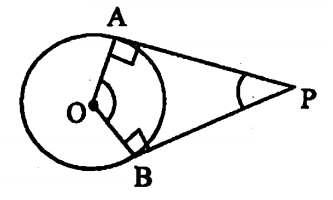Solution:

Given,

PA and PB are the tangents to the circle.

We know that radius is perpendicular to the tangent through the point of contact.

∠OAP = ∠OBP = 90°

∠OAP + ∠APB + ∠OBP + ∠AOB = 360°

90° + ∠APB + 90° + ∠AOB = 360°

∠APB + ∠AOB = 360° – (90° + 90°)

∠APB + ∠AOB = 360° – 180°

∠APB + ∠AOB = 180°

Hence proved.

OR

Prove that the sum of the squares of the sides of a rhombus is equal to the sum of the squares of its diagonals.

Solution:

Given,

ABCD is the rhombus in which diagonals AC and BD intersect each other at O.

To prove: (AB² + BC² + CD² + DA²) = AC² + BD²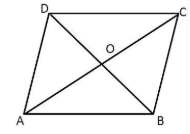Proof:

OA = 1/2 AC

OB = 1/2 BD

In right triangle AOB,

AB² = OA² + OB²

AB2 = (1/2 AC)2 + (1/2 BD)2

AB2 = 1/4 (AC2 + BD2)

4AB2 = AC2 + BD2

AB² + AB² + AB² + AB² = AC² + BD²

AB² + BC² + CD² + DA² = AC² + BD² (sides are equal in rhombus)

Hence proved.

20. Find the coordinates of the point which divides the join of (-1, 7) and (4, -3) in the ratio 2 : 3.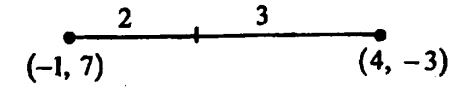Solution:

Let P(x, y) be the point which divides the line segment joining the points (-1, 7) and (4, -3) in the ratio 2 : 3.

(x1, y1) = (-1, 7)

(x2, y2) = (4, -3)

m : n = 2 : 3

Using section formula,

P(x, y) = [(mx2 + nx1)/ (m + n), (my2 + ny1)/ (m + n)]

= [(8 – 3)/(2 + 3), (-6 + 21)/(2 + 3)]

= (5/5, 15/5)

= (1, 3)

Hence, the required point is (1, 3).

OR

Find the value of y for which the distance between the points P(2, -3) and Q(10, y) is 10 units.

Solution:

Given,

P(2, -3) and Q(10, y)

PQ = 10 units

PQ2 = (10)2

(10 – 2)2 + (y + 3)2 = 100

(8)2 + y2 + 6y + 9 – 100 = 0

y2 + 6y – 91 + 64 = 0

y2 +6y – 27 = 0

y2 + 9y – 3y – 27 = 0

y(y + 9) – 3(y + 9) = 0

(y – 3)(y + 9) = 0

y = 3, y = -9

21. Match the following:

(i) sin (90° – A) (a) sin A

(ii) cos 0° (b) 0

(iii) sin 0° (c) 1

(iv) cos (90° – A) (d) cos A

Solution:

(i) sin (90° – A) = (d) cos A

(ii) cos 0° = (c) 1

(iii) sin 0° = (b) 0

(iv) cos (90° – A) = (a) sin A

OR

If 3 cot A = 4, check whether (1 – tan2A)/ (1 + tan2A) = cos2A – sin2A or not.

Solution:

Given,

3 cot A = 4

cot A = 4/3

tan A = 1/cot A = 1/ (4/3) = ¾

tan2A = (¾)2 = 9/16

(1 – tan2A)/ (1 + tan2A) = [1 – (9/16)]/ [1 + (9/16)]

= (16 – 9)/ (16 + 9)

= 7/25

Now,

cot A = side adjacent to A/side opposite to A = 4/3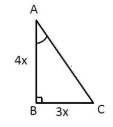By Pythagoras theorem,

AC2 = AB2 + BC2

= (4x)2 + (3x)2

= 16x2 + 9x2

= 25x2

AC = 5x

sin A = 3x/5x = ⅗

cos A = 4x/5x = 4/5

cos2A – sin2A = (⅘)2 – (⅗)2

= (16 – 9)/25

= 7/25

22. A kite is flying at a height of 60 m above the ground. The string attached to the kite is temporarily tied to a point on the ground. The inclination of the string with the ground is 60°. Find the length of the string, assuming that there is no slack in the string.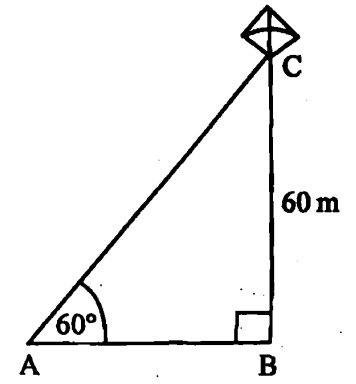Solution:

From the given,

AC = Length of the string.

In right triangle ABC,

sin 60° = BC/AC

√3/2 = 60/AC

AC = (60 × 2)/3

= 120/√3

= 40√3 m

23. Draw a circle of radius 3 cm. Take a point outside the circle. Construct the pair of tangents from this point to the circle.

Solution: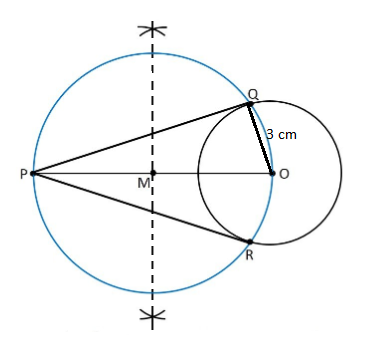Hence, PQ and PR are the required tangents to the circle.

24. In a circle of radius 21 cm, an arc subtends an angle of 60° at the centre. Find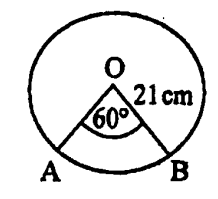(i) the length of the arc

(ii) area of the sector formed by the arc

Solution:

Given,

Radius = r = 21 cm

Angle of the sector = θ = 60°

(i) Length of an arc = (θ/360°) × 2πr

= (60°/360°) × 2 × (22/7) × 21

= (1/6) × 2 × (22/7) × 21

= 22 cm

(ii) Area of the sector = (θ/360°) × πr2

= (60°/360°) × (22/7) × 21 × 21

= 22 × 21

= 231 cm2

PART – D

25. Check graphically whether the pair of equations x + 3y = 6 and 2x – 3y = 12 is consistent. If so, solve them graphically.

Solution:

Given,

x + 3y = 6

2x – 3y = 12

Comparing with the standard form a1x + b1y + c1 = 0 and a2x + b2y + c2 = 0,

a1 = 1, b1 = 3, c1 = -6

a2 = 2, b2 = -3, c2 = 12

a1/a2 = ½

b1/b2 = 3/-3 = -1

a1/a2 ≠ b1/b2

Thus, the given system of equations has a unique solution.

Consider the first equation:

x + 3y = 6

3y = -x + 6

y = -(⅓)x + 2

 x -6 -3 0 6 y 4 5 2 0

Now, consider another equation:

2x – 3y = 12

3y = 2x – 12

y = (⅔)x – 4

 x -3 0 3 6 y -6 -4 -2 0

Graph: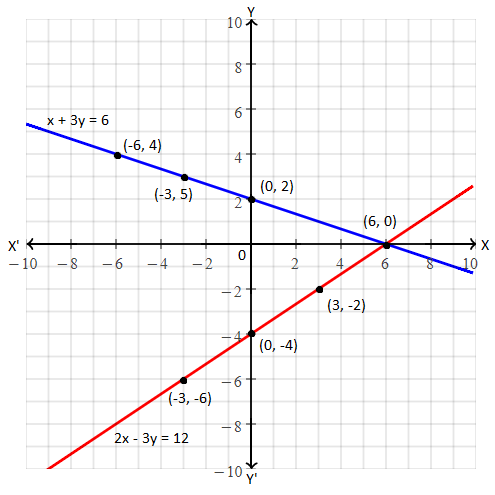Hence, the solution of the given pair of equations is x = 6 and y = 0.

OR

2 women and 5 men can together finish an embroidery work in 4 days, while 3 women and 6 men can finish it in 3 days. Find the time taken by 1 woman alone to finish the work, and also that taken by 1 man alone.

Solution:

Let x be the number of days taken by 1 woman to complete the work.

Let y be the number of days taken by 1 man to complete the work.

Thus,

Work done by 1 woman in 1 day = 1/x

Work done by 1 man in 1 day = 1/y

According to the given,

4[(2/x) + (5/y)] = 1

(8/x) + (20/y) = 1….(i)

And

3[(3/x) + (6/y)] = 1

(9/x) + (18/y) = 1….(ii)

(i) × 9 – (ii) × 8,

(72/x) + (180/y) – (72/x) – (114/y) = 9 – 8

(180 – 144)/y = 1

y = 36

Substituting y = 36 in (ii),

(9/x) + (18/36) = 1

9/x = 1 – (1/2)

9/x = 1/2

x = 18

Hence, the time taken by 1 woman alone to finish the work is 18 days and the time taken by 1 man alone is 36 days.

26. If a line is drawn parallel to one side of a triangle to intersect the other two sides in distinct points, the other two sides are divided in the same ratio. Prove it.

Solution:

Let ABC be the triangle and DE || BC.

Join BE and CD and then draw DM ⊥ AC and EN ⊥ AB.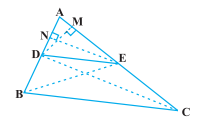ar(ΔBDE) = (1/2) × DB × EN

Also,

ar(ΔADE) = (1/2) × AE × DM

ar(ΔDEC) = (1/2) × EC × DM

Now,

ar(ΔADE)/ar(ΔDEC) = [(1/2) × AE × DM]/ [(1/2) × EC × DM] = AE/EC….(ii)

∆BDE and ΔDEC are on the same base DE and between the same parallels

BC and DE.

ar(ΔBDE) = ar(ΔDEC)….(iii)

From (i), (ii) and (iii),

Hence proved.

OR

Two tangents TP and TQ are drawn to a circle with a centre O from an external point T. Prove that ∠PTQ = 2∠OPQ.

Solution:

Given,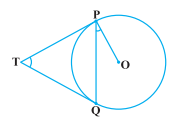Let ∠PTQ = θ

We know that TP = TQ

Thus, TPQ is an isosceles triangle.

∠TPQ = ∠TQP = 1/2 (180° – θ) = 90° – θ/2

Also, ∠OPT = 90°

∠OPQ = ∠OPT – ∠TPQ

= 90° – (90° – θ/2)

= θ/2

= 1/2 ∠PTQ

∠PTQ = 2∠OPQ

27. A solid iron pole consists of a cylinder of height 220 cm and base diameter 24 cm, which is surmounted by another cylinder of height 60 cm and radius 8 cm. Find the mass of the pole, given that 1 cm3 of iron has approximately 8g mass. (use π = 3.14)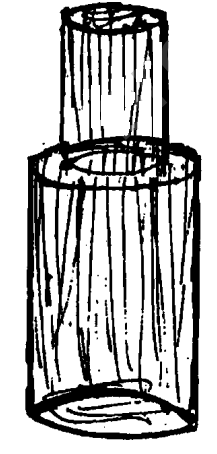Solution:

Given,

Radius of larger cylinder = R = 12 cm

Height of the larger cylinder = 220 cm

Radius of smaller cylinder = r = 8 cm

Height of the smaller cylinder = h = 60 cm

Volume of pole = Volume of larger cylinder + Volume of smaller cylinder

= πR2H + πr2h

= π[12 × 12 × 220 + 8 × 8 × 60]

= 3.14 (144 × 220 + 64 × 60]

= 3.14 × 35520

= 111532.8 cm3

Mass of 1 cm3 of iron = 8 g (given)

The mass of 111532.8 cm3 = 111532.8 × 8

= 892262.4 g

= 892.262 kg

OR

A wooden article was made by scooping out a hemisphere from each end of a solid cylinder as shown in the figure. If the height of the cylinder is 10 cm and its base is of radius 3.5 cm, find the total surface area of the article.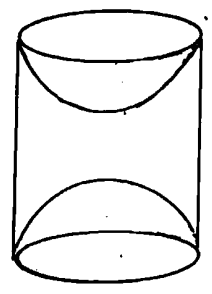Solution:

Given,

Radius of hemispherical part = Radius of cylindrical part = r = 3.5 m

Height of cylinder = h = 10 cm

Total surface area of the article = CSA of cylinder + CSA of two hemispheres

= 2πrh + 2 × 2πr2

= 2π[3.5 × 10 + 2 × 3.5 × 3.5]

= 2π[35 + 24.5]

= 2 × (22/7) × 59.5

= 44 × 8.5

= 374 cm2

28. A survey regarding the heights (in cm) of 51 girls of Class X of a school was conducted and the following data was obtained.

 Height (in cm) Number of girls Less than 140 4 Less than 145 11 Less than 150 29 Less than 155 40 Less than 160 46 Less than 165 51

Find the median height.

Solution:

The given data can be tabulated as below:

 Height (in cm) Number of girls (fi) Cumulative frequency 0 – 140 4 4 140 – 145 7 11 145 – 150 18 29 150 – 155 11 40 155 – 160 6 46 160 – 165 5 51

n/2 = 51/2 = 25.5

The cumulative frequency greater than 25.5 is 29 which lies in the class 145 – 150

l = 145

cf = 11

f = 18

h = 5

Median = l + {[(n/2) – cf]/ f} × h

= 145 + [(25.5 – 11)/18] × 5

= 145 + (72.5/18)

= 145 + 4.03

= 149.03

Hence, the median height is 149.03 cm.

OR

The following data gives the information on the observed lifetime (in hours) of 225 electrical components:

 Lifetime (in hours) 0 – 20 20 – 40 40 – 60 60 – 80 80 – 100 100 – 120 Frequency 10 35 52 61 38 29

Determine the modal lifetimes of the components.

Solution:

From the given,

Maximum frequency is 61 which lies in the class interval 60 – 80.

Modal class = 60 – 80

Lower limit of the modal class = l = 60

Frequency of the modal class = f1 = 61

Frequency of the class preceding the modal class = f0 = 52

Frequency of the class succeeding the modal class = f2 = 38

Class height = h = 20

Mode = l + [(f1 – f0)/ (2f1 – f0 – f2)] × h

= 60 + [(61 – 52)/ (2 × 61 – 52 – 38)] × 20

= 60 + [9/ (122 – 90)] × 20

= 60 + (9/32) × 20

= 60 + 5.625

= 65.625

Hence, the modal lifetimes of the components is 65.625 hours.